# 2.10 – Lesson 2 Summary

Previous: 2.9 – Example

## Relationship Between CDF and PDF

One of the key features of a random variable is its associated probability distribution, which gives the probabilities that we can observe a certain event, or set of values, under the given random variable. This distribution can take the form of either a cumulative distribution function (CDF) or a probability density function (PDF). For continuous random variables, these two functions are related by the Fundamental Theorem of Calculus: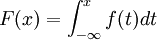The integrand is the PDF of our continuous random variable, and the corresponding integral is the CDF.

## Calculating Probabilities

These two functions give the probabilities associated with observing certain events under a random variable X in question. The CDF has a direct probabilistic interpretation, given by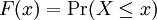Using the relationship between the CDF and the PDF, probabilities for events associated to continuous random variables can be computed in two equivalent ways. Suppose we wish to calculate the probability that a continuous random variable X is between two values a and b. We could use the PDF and integrate to find this probability.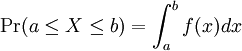Alternatively, if we wish to use the CDF, we can evaluate the difference F(b) - F(a) to find this probability.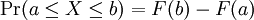Of course we know that both approaches yield the same result. This fact is precisely the statement of the Fundamental Theorem of Calculus.

## Expected Value, Variance and Standard Deviation

The expectation represents the "centre" of a random variable, an expected value of an experiment, or the average outcome of an experiment repeated many times. The variance of a random variable is a numerical measure of the spread, or dispersion, of the PDF of X. Given the PDF f(x) of a continuous random variable X, students should know: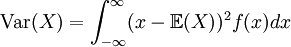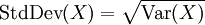Note that the standard deviation of X is sometimes denoted σ(X).

Previous: 2.9 – Example